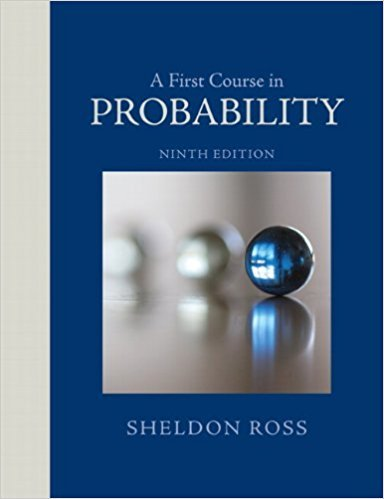×
Get Full Access to A First Course In Probability - 9 Edition - Chapter 3 - Problem 6p
Get Full Access to A First Course In Probability - 9 Edition - Chapter 3 - Problem 6p

×

# Consider an urn containing 12 balls, of which 8 are white.ISBN: 9780321794772 63

## Solution for problem 6P Chapter 3

A First Course in Probability | 9th Edition

• Textbook Solutions
• 2901 Step-by-step solutions solved by professors and subject experts
• Get 24/7 help from StudySoup virtual teaching assistantsA First Course in Probability | 9th Edition

4 5 1 385 Reviews
19
4
Problem 6P

Problem 6P

Consider an urn containing 12 balls, of which 8 are white. A sample of size 4 is to be drawn with replacement (without replacement). What is the conditional probability (in each case) that the first and third balls drawn will be white given that the sample drawn contains exactly 3 white balls?

Step-by-Step Solution:

Solution :

Step 1 of 1:

Given the total balls is 12 and 8 are white balls.

Then the sample size is 4.

Our goal is:

We need to find the conditional probability (in each case) that the first and third balls drawn will be white given that the sample drawn contains exactly 3 white balls.

Let F denotes the event that the first and third drawn balls are white and

Let E denotes the event that the sample contains exactly 3 white balls.

Then we desire to compute

P(F/E) =P(E) =P(E) =P(E) =And P(FE) is the probability that our sample has 3 white balls and the first and and third balls are white.

Here to conclude this we can explicitly enumerate the possibilities in FE as {(W,W,W,B), (W,B,W,W)}, showing that

P(FE) =Given these 2 results we have that

P(F/E) =P(F/E) =P(F/E) =P(F/E) =To work the problem with replacement we have that

P(E) =P(E) =Before we enumerated the sample in EF, this set is

{(W,W,W,B),(W,B,W,W)} and has probabilities is given byStep 2 of 1

##### ISBN: 9780321794772

Unlock Textbook Solution